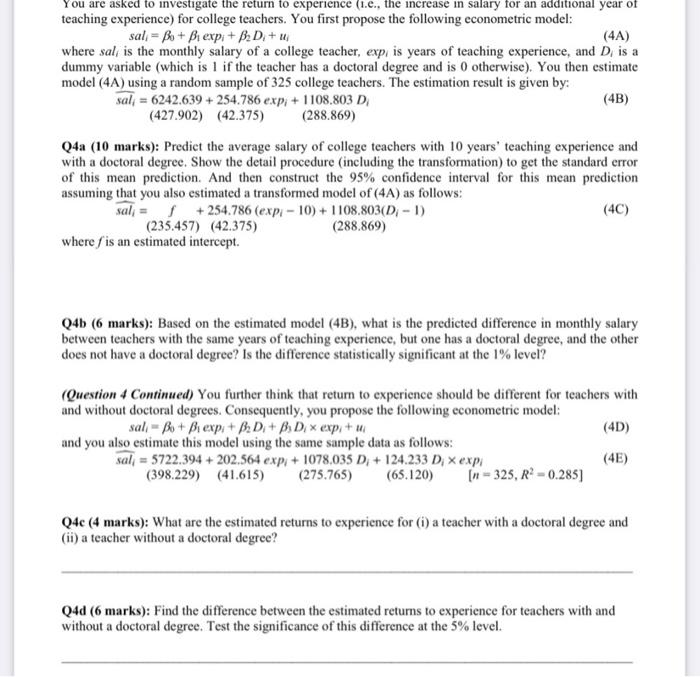Question

# You are asked to investigate the return to experience (1.e., the increase in salary for an additional year of teaching experience) for college teachers. You first propose the following econometric model: sal = Bo + Bi expi+ B, D + u (4A) where sal, is theYou are asked to investigate the return to experience (1.e., the increase in salary for an additional year of teaching experience) for college teachers. You first propose the following econometric model: sal = Bo + Bi expi+ B, D + u (4A) where sal, is the monthly salary of a college teacher, exp, is years of teaching experience, and D, is a dummy variable (which is 1 if the teacher has a doctoral degree and is otherwise). You then estimate model (4A) using a random sample of 325 college teachers. The estimation result is given by: sal = 6242.639 + 254.786 exp;+ 1108.803 D (4B) (427.902) (42.375) (288.869) Q4a (10 marks): Predict the average salary of college teachers with 10 years' teaching experience and with a doctoral degree. Show the detail procedure (including the transformation) to get the standard error of this mean prediction. And then construct the 95% confidence interval for this mean prediction assuming that you also estimated a transformed model of (4A) as follows: sal = l +254.786 (expi-10) + 1108.803(D, -1) (4C) (235.457) (42.375) (288.869) where ſis an estimated intercept. Q4b (6 marks): Based on the estimated model (4B), what is the predicted difference in monthly salary between teachers with the same years of teaching experience, but one has a doctoral degree, and the other does not have a doctoral degree? Is the difference statistically significant at the 1% level? (Question 4 Continued) You further think that return to experience should be different for teachers with and without doctoral degrees. Consequently, you propose the following econometric

Q4 a) 10 years teaching experience; Doctoral degree

10* (254.786) + 1108.803 = 3656.663

3656.663 + Intercept

3656.663 + 6242.639 = 9899.302

95% Confidence Interval

9899.302 (+ / -) (1.96* 427.902) (+ / -) (254.786* 1.96* 10*42.375) (+ / -)( 288.869*1.96)

= 9899.302 (+ / -) (213017.38)

= ( -203118.08, 222916.68546)

b) the difference is 1108.803.

Coefficient / Standard error = 1108.803 / 288.869 = 3.8384

t(324, 0.01) = 2.326

t-statistic is greater than the t-critical value. Null hypothesis is rejected.

Alternative hypothesis: The value statistically different from zero.

c) Without a doctoral degree; the change for one year of experience = 202.564

Addition for teachers with a doctoral degree = 202.564 + 124.233 = 326.894

d) Difference for a doctoral degree = 124.233 / 65.122 = 1.9076

t(324, 0.05) = 1.645

t-statistic is greater than t-critical.

Null hypothesis of the coefficient for the Doctoral being zero is rejected.

Alternative hypothesis: The coefficient is statistically different from zero.

The coefficient is statistically different from zero.

#### Earn Coins

Coins can be redeemed for fabulous gifts.